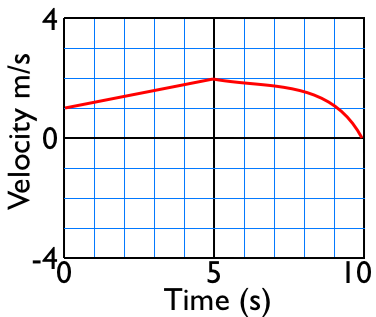Answer these questions on a seperate sheet of paper.
 For the v vs t graph, how do you find: (1) The velocity at 4 seconds? (2) If the acceleration is positive or negative? (help) (3) The displacement from 1 to 3 seconds? (help) (4) The change in position from 1 to 3 seconds? (help) (5) Is the velocity is positive or negative at 3 seconds? (6)The velocity at 9 seconds?For the x vs t graph, how do you find: (7) The velocity at 4 seconds? (help) (8) If the acceleration is positive or negative? (9) the displacement from 1 to 3 seconds? (10) the change in position from 1 to 3 seconds? (11) if the velocity is positive or negative at 3 seconds? (help) (12) the velocity at 9 seconds? (help)Notice how the questions are the same for each section but how they are answered is different because you are given a different graph for each question set.

by Tony Wayne ...(If you are a teacher, please feel free to use these resources in your teaching.)